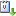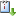Cuándo 05/09/2016 de 17:00 a 18:00 Salon de seminarios Graciela SalicrupvCaliCal
In this talk we will show that in certain spacetimes with hypersurface and stringlike singularities on still has local well-posedness of the wave equation in the Sobolev space $$H^1$$. This function space is chosen as it allows us to define the energy-momentum tensor of the field distributionally. It is also une needed for solutios of the linearised Einstein equations. The methods we employ are therefore also relevant to finding low regularity solutions of the Einstein equatiosn which, as shown by Dafermos, is an important issue when considering Cosmic Censorship.
Motivated by this work we propose a definition of a strong gravitational singularity as an obstruction to the evolution of a test (scalar) field rather than the usual definition as an obstruction to the evolution of a test particle along a casual geodesic. This definition has the advantage that it is directly related to the physical effect to the singularity on the field (the energy-momentum tensor fails to be integrable) and also that it may be applied to situations where the regularity of the metric falls below $$C^{1,1}$$ where one no longer has existence and uniqueness of geodesics.